Fibonacci Analysis by Mark Deaton

Fibonacci Analysis is the single best way to predict price action.

Through careful assesment of a longer time frame then the one you are trading you can quickly identify where price will go to next and where it will likely encounter resistance and reverse.

The proper way to implement Fibonacci analysis is by means of Fibonacci retracements and extensions. These terms describe the two means of forecasting price action horizontally speaking. Truthfully this is the only way I use Fibonacci anyway.

Horizontally, meaning horizontal lines across your chart. A retracement is when price starts at a low or high then makes a new low or high and then begins to correct. The corrective wave is the retracement you are measuring and this type of Fibonacci analysis is measuring the move back to the start.
In other words if price makes it all the way back we would say that price has retraced 100%.

If however price makes it just halfway back we would say price has retraced how much?

Thatâ€™s right 50%.

Lets look at a 50% retracement… In the image below price starts at 100% comes down to 0% and begins to rise – retracing its way back to 0%. Understand we didn’t lay down the Fibonacci levels until AFTER we reached 0% and began to retrace then and only then do we do our Fibonacci Analysis. Notice at the 50% level price encounters serious resistance and fails to penetrate.Your Fibonacci analysis should consist of learning how to properly identify Fibonacci retracements as well as Fibonacci extensions and thats it. These levels are your key to forecasting high probability price reversals and continuations.

When you begin to analyze your chart using Fibonacci analysis understand that what you are looking for is simple confluence. The heart of Fibonacci is really nothing more than support and resistance levels that have demonstrated hesitation or reversals at these levels you are looking at now.

The more you see in the past that concides with your current analysis the more you will realize that your analysis is accurate. If the only time price is hitting a Fibonacci line is right now, then your analysis is likely innacurate.

So when you are doing your Fibonacci analysis make sure that the area you have identified as a retracement level is also a level that has been tested in the past and proven to be an important level.

Your job is to identify high odds places on the chart so you need this kind of agreement otherwise you may as well flip coins.Author:
Mark Deaton
www.Fibonaccigenius.com

Fibonacci Retracements

The Fibonacci Retracement is probably the most heavily used Fibonacci tool in the toolset. You will find Fibonacci Retracements as a solid tool in identifying key support and resistance areas.

If prices have fallen from a recent swing high down to a swing low, the expectation is that price should retrace distance, high to low, by a ratio of the Fibonacci sequence.

You can use Fibonacci retracements and extension from a tick chart through a daily, monthly and weekly. Literally any time frame.
It is important to note, the larger price move from swing high to swing low, the more accurate the retracement projections. Identification and selection of the correct swing points are keys to success.

While there are many variations of the ratio set, simple is better, lets focus on four major retracement levels.
â€¢ 23.6% — The shallowest of the retracements. In very strong trending markets price typically quickly bounces in the area of this ratio.
â€¢ 38.2% — This is the first line of defense of the current trend. Breaking this level starts to erode the underlying trend.
â€¢ 50% — The neutral point of any retracement. This is the critical tipping point.
â€¢ 61.8% — retracing to this typically signals a breakdown in the trend.
â€¢ 100% — Matching the move

In this section we will also show examples of how potential opportunities form when price retraces beyond 100% by following another set of Fibonacci ratios:
â€¢ 138.2%
â€¢ 161.8%
â€¢ 200%

Notice in each case we have simply added 100% to the standard ratio set. I use this set of retracements on a daily basis, from 23.6% all the way to 200% and sometimes 300% For my style of trading I find 38.2%, 50% and 61.8% quite reliable.I use the other primarily as confirmation levels.

So lets take a look at some examples of Fibonacci Retracements in use.

Example 1:

Take the example below. The EUR/USD had risen from 1.3360 to 1.4278. The next day the EURUSD failed to make a new high and the potential swing point was in place. So I using swing points I placed a Fibonacci retracement on my chart.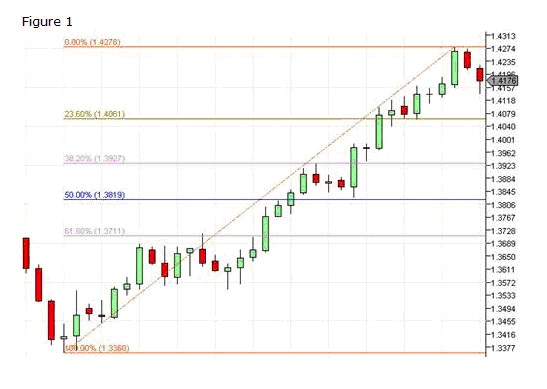The trend was obviously very strong and the first retracement to the 23.6% level was met with a violent change in direction. You can see the dip below the 23.6% level and the sudden reversal. While there are multiple entry methods, the most conservative would be to wait until the level is penetrated and price establishes itself above that level and enter on the open of the next bar as shown.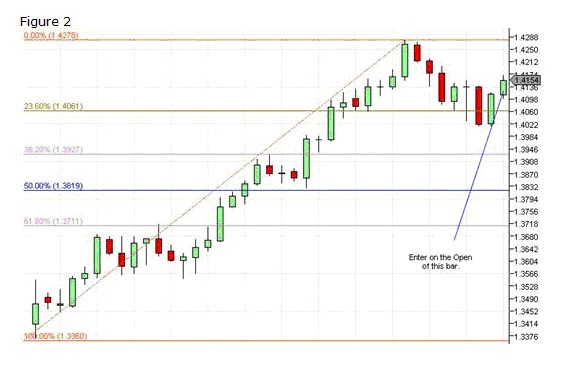With the right money management, you can see in this example this could have been a serious winner.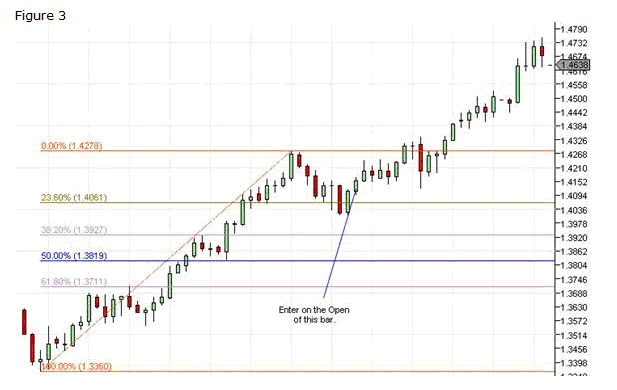Once you understand the method you can find countless examples. Every market, FOREX, Equities and Futures each exhibit these patterns to some degree.

Example 2:
Lets look at another example using the USDCAD. You can see in this example there are multiple entry points for both trend and countertrend trades.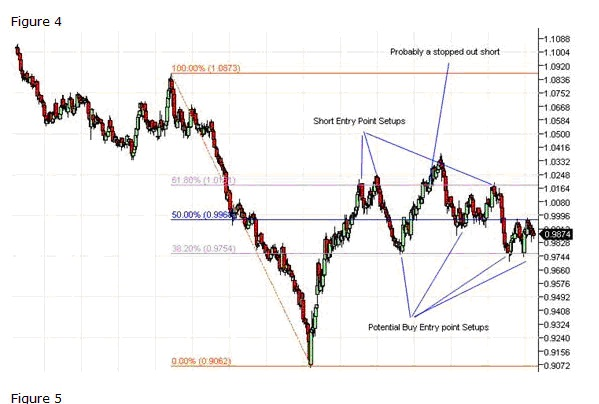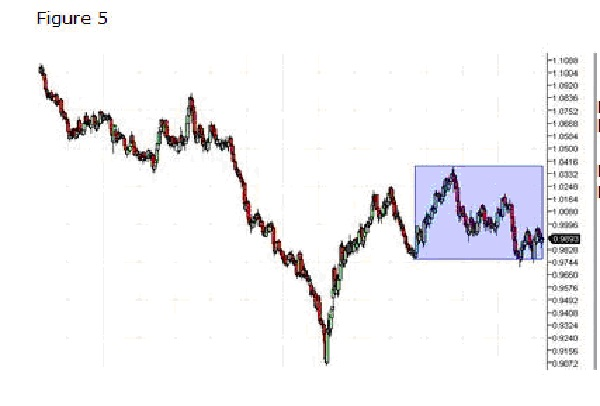Lets zoom in and look at the area highlighted in blue. Fibonacci Ratios work on virtually any size price swing.
The chart below shows the Fibonacci Retracement applied to the smaller price swing.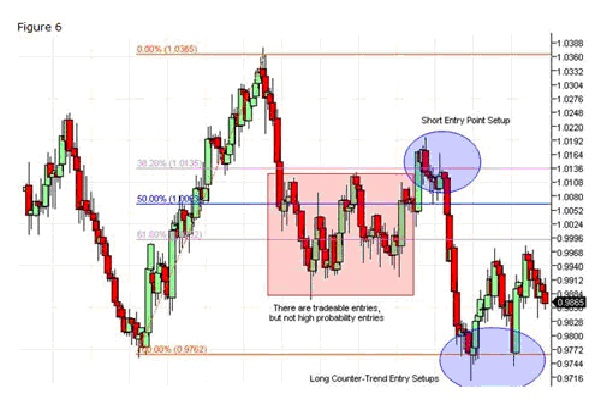The blue ellipses show the high potential entry points. Notice, in each of these cases you could have entered the market with a relatively tight stop loss with high reward potential.

Ok, we have shown some examples of well behaved price action. What happens if price retraces 100%? How far can it go beyond this point? Fibonacci ratios provide some clues to answering this question and finding low risk entry points.

Example 3:
The example below shows the GBPUSD making a bottom and bouncing back. And multiple entry points from the same set Fibonacci Retracement levels.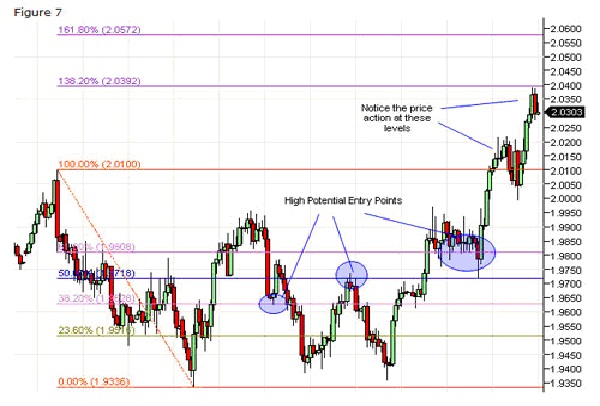Of note are the high potential entry points at 38.2%, 50% and 61.8%. Each of these could have been entry points with solid profit potential. However, notice after the initial breakout above 100%, there were other opportunities to get in the trade. Ultimately price jumped to the 138% point before backtracking.

This example shows yet another way to use Fibonacci Retracements. This example shows why it is valuable to identify potential levels above and beyond the initial 100% retracement.

Retracements are the cornerstone of Fibonacci theory as it applies to the financial markets. Hopefully these examples have provided guidance from which to draw your own retracements and expand your trading toolset.

To recap, while there are other retracement values, my defaults Fibonacci Retracements always include: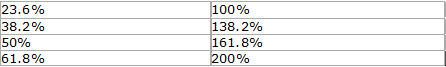You can never tell when price action it going well beyond the 100% level.

Author:

Mark Deaton
www.Fibonaccigenius.com

Posted in Uncategorized | 1 Reply

Fibonacci Trading is not difficult. If you are new to using Fibonacci tools then look around this site and be sure to download our free manual and Fibonacci trading system.

The system is simply a tool that you can use to begin to learn just how you can use Fibonacci trading tactics in your overall trading strategy.

Fibonacci trading simply involves measuring swing highs and lows and attempting to forecast price reversals and continuations.

Fibonacci trading concepts can get complicated, the story and science behind it can get fascinating and even a bit overwhelming. If you want to learn about the theories and science behind Fibonacci trading concepts you are at the wrong web site.

If however you want step by step instructions on how you can begin to apply the trading principles using Fibonacci then you have the arrived.When price makes its way in any direction up or down a Fibonacci retracement measures its mark back to where it started. So lets say price started at 40.00 and then went to 50. Thatâ€™s a 10.00 move right?

Well generally speaking after a move like that there tends to be a degree of profit taking from traders. People are going to start selling off and cashing in on the move.

These moves and profit taking scenarios are what causes the markets to move up and down. Generally in a bull market you will see a large move up, a retracement profit taking period and then a new thrust up once again.
The market stays bullish as long as the profit taking doesn’t meet or exceed a 100% retracement.
For example a \$10.00 move up in a week, then a \$5.00 retracement the next is a 50% retracement.
If price moves up \$10.00 again then we are making bullish progress. \$40 to \$50 is a \$10 dollar move. A pullback or retracement to \$45 is a 50% retracement. A new \$10 dollar move up is a target of \$55.

So in time we are making our way up in a fashion that is as old as time itself.
These Fibonacci trading principles are called retracements.

Even if price were making 76% retracements we are still making bullish progress. Weak progress but bullish none-the-less.

In a super strong bull market a retracement as little as 23.6% is commonplace.

So you see the degree of retracement can tell you much about market strength.

Lastly anything beyond a 100% retracement is called a Fibonacci extension.

Author:
Mark Deaton
www.Fibonaccigenius.com

Fibonacci explained- By Mark Deaton

Fibonacci explained - the following is a breakdown of how you can use Fibonacci in your stock, options and forex trading.
The Fibonacci sequence is a mathematical sequence in which each number, after two starting values, is the sum of the two preceding numbers. The sequence runs:
0, 1, 1, 2, 3, 5, 8, 13, 21, 34, 55, 89, 144, 233, 377, 610, 987, 1597â€¦â€¦â€¦

Dividing a Fibonacci number by its immediate predecessor yields an approximation of the Golden Ratio (roughly 1.6180327868852). The higher the Fibonacci number, the closer the approximation to the Golden Ratio becomes.

The sequence is named for medieval mathematician Leonardo of Pisa, commonly known as Fibonacci, who was responsible for introducing the Hindu-Arabic numeral system we currently use to Europe. Prior to Fibonacci, Roman numerals held sway.

Fibonacciâ€™s namesake sequence stems from his solution to the problem of modeling the growth of rabbit populations under ideal conditions, although it has since been used in many other contexts.

Today Fibonacci numbers are used extensively in the study of mathematics, computer science and biology. The Golden Ratio shows up music, art and architecture and is often used as a guide for creating visually pleasing proportions.

Perhaps because it is relatively easy to understand, the Fibonacci sequence is also frequently referenced in pop culture. For example, it makes a prominent appearance in The DaVinci Code as the password that opens the codex.

In finance, Fibonacci numbers, or more precisely the Golden Ratio, form the basis of a popular method of technical analysis. The idea is that in the aftermath of a significant price movement, subsequent levels of support and resistance will form around â€˜Fibonacci Significantâ€™ numbers, usually 38.2%, 50% and 61.8%, which are percentages of the total price drop or gain.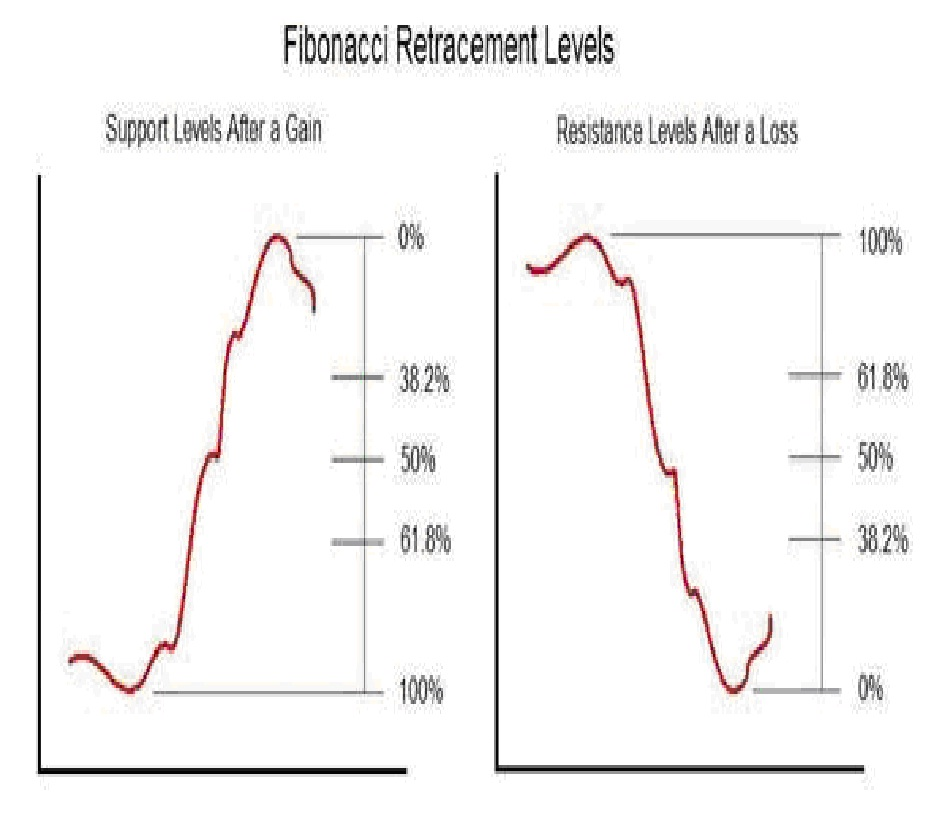After a large price movement, technical traders pay particular attention to these retracement levels. For example, if the price of a stock has recently shot up, a technical trader might sell until the price declined to the 38.2% level and begin buying when it reached the 61.8% level.
Conversely, after a big decline, the same trader might buy until the price recovered to the 38.2% level and begin selling as the price approached the 61.8% level.

Fibonacci Extensions are, appropriately enough, an extension of the same idea. Technical traders have observed that after a period of retracement, stock prices often resume moving in accordance with their original trend.
Extending the Fibonacci analysis allows technical traders to predict the next turning point. Fibonacci Extension levels are calculated based on the original price movement.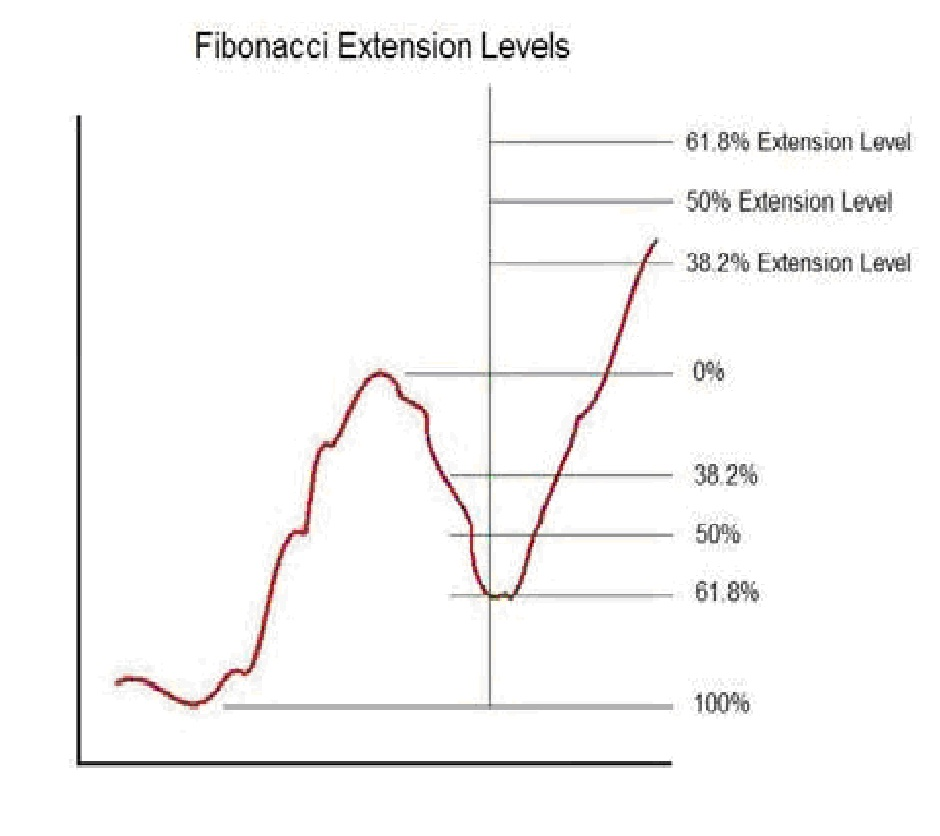Having observed a major price increase in a stock, a technical trader might begin buying when the price had subsequently declined to the 61.8% level, then hold the stock until the price approached the 61.8% extension level, at which point it would be time to sell in anticipation of a new retracement. Extensions are applied in a similar manner for price declines, with the expectation being that after a retracement, the price decline will resume.

Fibonacci Effectiveness
There is not any strictly rational reason why stock prices should behave as Fibonacci analysis predicts. While it is true that the Golden Ratio appears frequently in nature, this does not in any way imply that we should expect it to play a role in financial markets. After all, rabbit population growth has very little to do with stock prices.

However, it would be a mistake to dismiss Fibonacci methods as useless superstition. The fact is that there are many active share traders who use Fibonacci retracements and extensions to guide their trading strategy.
If enough traders use and act on Fibonacci analysis, the method will work, regardless of whether or not it has any rational basis. In the short term at least, even ill founded theories can move markets. Regardless of whether or not Fibonacci naturally influences the market, the use of this analysis by many traders leads to an overall self-fulfilling prophecy in stock prices.

Phenomena like this are not uncommon in markets, and in fact, market psychology is a major focus of study in the field of Behavioral Economics.
In essence, while Fibonacci retracements and extensions may not have any real basis from a strict financial analysis perspective, they are a useful tool for predicting the behavior of many traders operating in the market.
For this reason, Fibonacci analysis can be an effective part of an overall trading strategy.

The key is to develop an understanding of how other traders are applying Fibonacci analysis. Target selection is also important. If past price movements of a stock appear to conform to Fibonacci predictions, then it is likely that traders using Fibonacci analysis are active in the trading of that particular stock.

This in turn improves the odds that Fibonacci analysis will be effective in predicting the future movements of that stock. Applied with a thorough understanding of how and where other traders are using it, Fibonacci retracements and extensions can be solid enhancer of trading profits.

Author
Mark Deaton
www.Fibonaccigenius.com

Posted in Uncategorized | 1 Reply

My Forex Fibonacci Trading System – Kelvin Lee

If you have read my previous post showing you how to plot the forex Fibonacci indicator on your chart, you will understand the power of the 0.382, 0.500 and the 0.618 Fibonacci retracement level. These retracement levels are areas where you will the price being repelled by them. What makes Fibonacci trading such a popular system is because of its ability to predict the subsequent movement of the price which is through its extension.
Here is how the extension works:

1) When you see the price retracing to the 0.618 level and then being repelled by it, there is a high chance that the price will extend itself to the 1.618 level.
2) When you see the price retracing itself to the 0.500 level and then being repelled by it, there is a high probability that you will see the price extends to the 1.500 level or even 1.618.
3) If you see the price retracing itself to the 0.382 level and then repelled by it, you will most probably see the price extend itself to the 1.272 level and then move to the 1.382 level.

With your understanding of the extension, you will now be able to understand how this forex Fibonacci trading system works.

First of all, you need to setup either the Stochastic or RSI to help to identify whether the market is oversold or overbought.

Next, you need to setup a MACD indicator to help you identify the right time for entry.

Once you got this 2 indicators setup, you will need to draw Fibonacci whenever you see a swing high and a swing low. All you need to do is to wait for the price to retrace back to either one of the 3 levels and then check your indicators for signal. If the price did not retrace but continue to move higher or lower, you just have to remove your Fibonacci and then redraw them again with the new swing highs or swing lows.

Whenever you see the price retracing near a level, you should check your indicators for the following

1) If you are in an uptrend and you see the price retracing back to the 0.500 level, you should check your Stochastic indicator to see if the market is oversold or not. If it is indeed oversold, you should then move on to see your MACD indicator and wait for the histogram to flip over to the upside again before you enter your trade.
After you have entered a trade, your exit strategy is equally important. You will usually exit your trade 10 pips before the expected extension and you should always place a stop loss around 20 to 30 pips below the level of retracement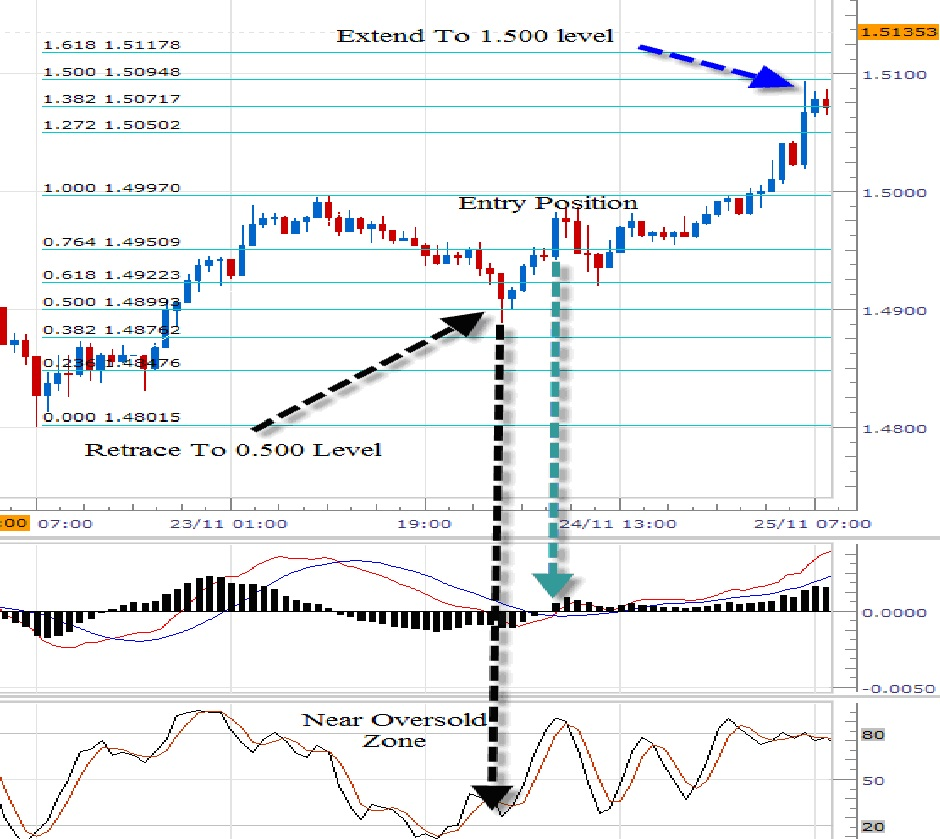2) If you are in a downtrend, the conditions that are stated above shall be reversed.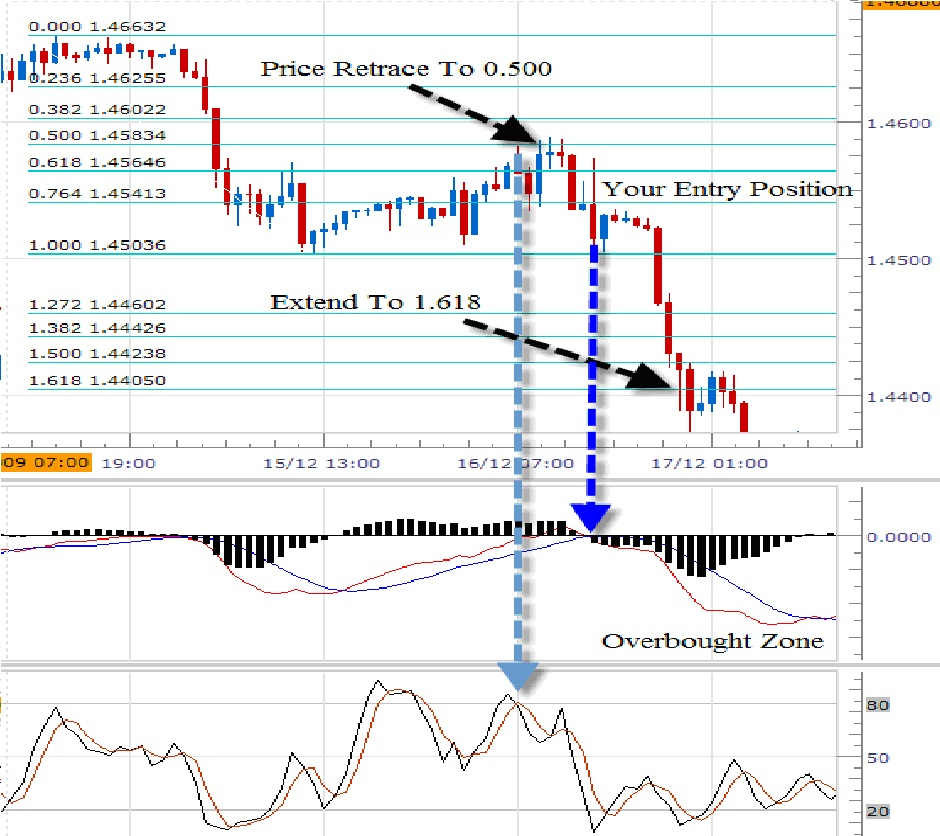If your Fibonacci retracement levels coincide with a major support or resistance level, this will give you more strength in that level and thus increase your chance of winning.
Do not start to trade immediately with this forex Fibonacci strategy. You should always try any new strategy out on your demo account and then move it to live only when you are able to trade profitable with it consistently.

Author:
Kelvin Lee
http://www.forexindicator.org

Forex Fibonacci Indicator Explained

As most of you know that the forex market basically moves in waves and there will be time where the market extends and there will also be time where the market retraces.

One of the best tools that you can use to time this retracement and extension is the forex Fibonacci levels.

So What Exactly Is Fibonacci?
It is a number sequences that is named after Leonardo of Pisa and the Fibonacci number sequences goes like this
0, 1, 1, 2, 3, 5, 8, 13 and so on. (Add the first 2 numbers to get the next)
However in trading, we are not interested in the sequences, we are actually interested in the Fibonacci ratio that the sequences create.

These are the ratio that we use as a forex trader.

Below are the retracement ratios
- 0.236
- 0.382
- 0.500
- 0.618
- 0.764
Below are the extension ratios
- 1.272
- 1.382
- 1.500
- 1.618

So How Can You Use These Ratios In Trading?
The Fibonacci ratio is in fact used as a level of support and resistance. These are areas where you willSELL or BUY depending on what you see and where you are.
Although there are quite a number of ratios given above, the important ones are the 0.382, 0.500, and 0.618 as they are usually area of strong support when the price retraces down and area of strong resistance when the price retraces up.

Here Is How You Should Plot Your Fibonacci Levels In An Uptrend:
Step 1: Using the tool provided by your platform, Pick a high point and a low point
Step 2: Select the levels that you want to display. (We will select 0.382, 0.500, 0.618, 1.272, 1.382, 1.500 and 1.618)
If you are in a downtrend, all you have to do is to switch the step 1 points.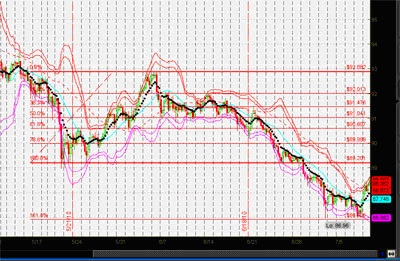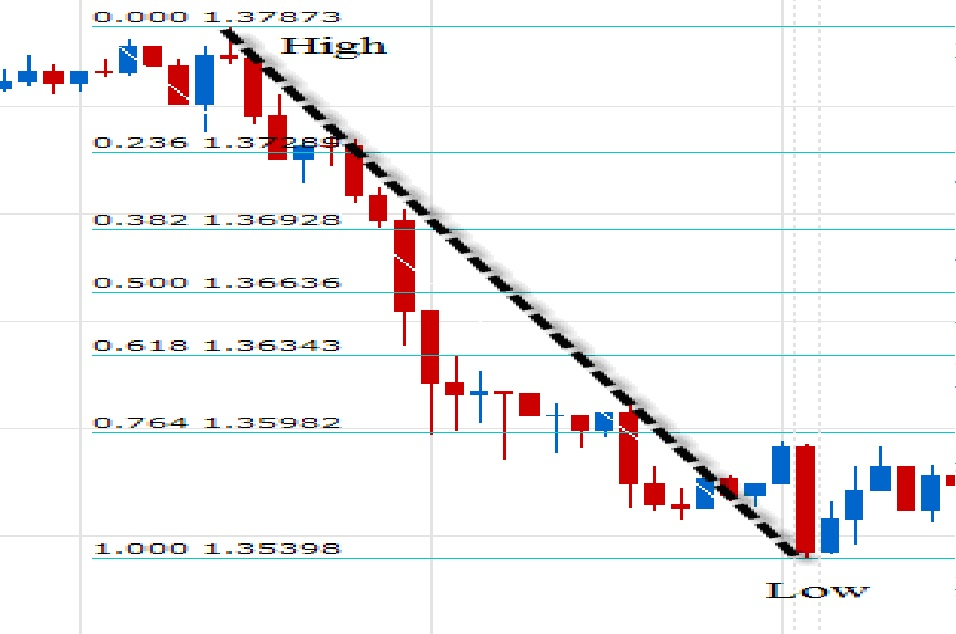In the case where you are in an uptrend, you will find that the retracement of your price will usually land on the 0.382, 0.500 or the 0.618 level as these are area of strong support and the price will then extend up to continue in its uptrend movement.
If you ever find the price moving below the 0.382 level, there is a high chance that the trend is reversing.

In the case where you are in a downtrend, the market will retrace upward and it will also find its resistance at 0.382, 0.500 and the 0.618 levels. Similar to the uptrend, if the market retraces above the 0.382 level, there is a high chance that the market is reversing.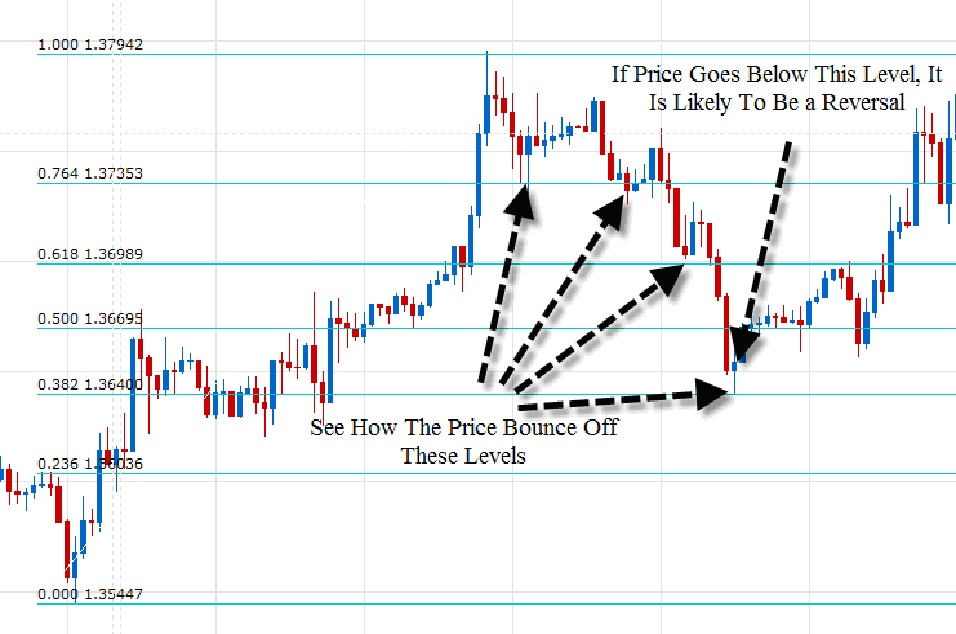In my next blog post, I will show you how to trade using Fibonacci Strategy and how you can make use of the 1.272, 1.382 and the 1.618 levels. In the meantime, you should try to plot the Fibonacci levels on your chart to see the power of it.

Author
Kelvin Lee
http://www.forexindicator.org